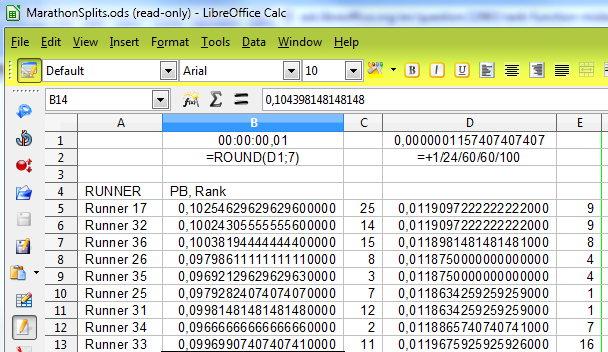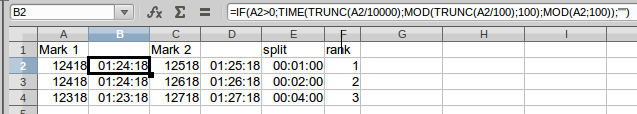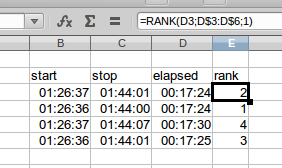# Rank function misbehaving with time values

I’m trying to rank split times from a marathon, but the RANK() function does not give equal rank to equal times. I’ve tried feeding the function absolute times (e.g. 18:15) and it works fine, but not when fed a calculated time (e.g. 15k time minus 10k time). Any thoughts.

Please clarify what “a calculated time (e.g. 15k time minus 10k time)” means. Does “15k” refer to a specific time or a distance run? If you could provide an example of the time displayed (and underlying number) that results from this calculation that would be helpful e.g., “18:15:00” displayed = 0.7604166667. Also please indicate your operating system and LO version.

The results of the race give the time for each runner at 5 kilometres, 10 kilometres, etc. I therefore, for example, subtract the 10k time from the 15k to get the time taken for that 5k split. The elapsed time column is formatted H:MM:SS and the split time column as MM:SS. I then wish to use the RANK() function to compare runners for each 5k split. I’m on 4.05 with Windows 7 and also on Ubuntu 10.4.

Later] I may have a clue: initially I laboriously typed times in as e.g. “1:24:18” and ranking worked correctly. I then added a column J allowing me to enter just 12418 and used the function IF(J2>0,TIME(TRUNC(J2/10000),MOD(TRUNC(J2/100),100),MOD(J2,100)),"") to get the time for column K. Ranking now gives different ranks to equal times.

Well, I think rounding is the key, we are dealing with the precision for decimal numbers and their binary representation.

Enter in a cell: 00:00:01
change the format to a number with 16 decimal positions: 0,0000115740740740

With operations without rounding we can have differences in the last numbers of the precision, that can change the RANK() between two numbers in appearance equals.

Round with seven decimal places must be enough to preserve up to hundredths.I think you and @horst are right. I can understand that rounding can be an issue in certain circumstances. Even so, I would expect a pair of date/time cells with “00:17:24”, which equates to the number 0.0120833333333333 (presumably repeating) to be equatable. Under v4.1.0.4 the number 0.0120833333333333 if resulting from a date is not equatable with itself, while if it is entered manually (as a number) it is equatable. A lot of people are going to consider that a bug.

Thanks for clarifying your situation. I can now reproduce the problem with the `RANK()` function here using v4.1.0.4 under Crunchbang 11. Initially, I used whole numbers converted to times in the manner you described, but this did not reveal any problem with the function. Here is a screenshot of this method:The split column is column D minus B. The formula in F2 is `=RANK(E2;E\$2:E\$4;1)` and this is copied to F3 and F4.

It appears that identical values are given the same rank, thus equal first placings are both denoted with “1”, and then the ranking skips to 3. If there are then equal third placings both will be denoted with a “3”, and then the ranking skips to 5, and so on. The LO help entry for this function does not mention how equal values are handled, however the AOO help page for the same function does indicate that there are “issues.”

The suggested AOO workaround (append `+COUNTIF(E\$2:E2;E2)-1` in my example) does produce unique values based on order of appearance. For example, if I copy the three rows shown in my example, so there are equal firsts, equal seconds, and equal thirds, (and expand the range from `E2:E4` to `E2:E7`) the results display as 1,3,5,1,3,5. If I then add the workaround to the formula (e.g., `=RANK(E2;E\$2:E\$4;1)+COUNTIF(E\$2:E2;E2)-1` for the first entry) then the results display as 1,3,5,2,4,6, which would seem better.

UPDATE: Using time values from your example file (refer comments below), in particular rows 26,32,33,38 and columns Z,AE,AG,AH, I can now reproduce the issue. In a new Calc file I manually keyed in the start (25k mark) and stop (30k mark) times, and used a simple subtraction of the start time from the stop time to obtain the elapsed time (5k split). A basic ranking of these elapsed times does not appear to give correct results as shown in this screenshot:The ranks IMO should show 1,1,4,3, yet for some reason the first two entries are determined to be different. We need to raise a bug and provide a simple example file like the one I have prepared. I will confirm this problem if you raise the bug. Please post the bug number back here in the format “fdo#123456” so that we can do this.

EDIT: Bug fdo#69068 has been raised.

That’s fine if the split times are all different. The problem occurs when some of the splits are the same.

If, say, the best three splits are the same they should be ranked 1,1,1 with the next best ranked 4. This sometimes happens.

However, sometimes (in the same sheet) these splits are producing 1,2,3,4. I even have a mixture of correct and incorrect: 1,2,2 for three equal values. Weird

Ok. Thanks for clarifying. I will update my answer.

Your 1,3,5,1,3,5 example is exactly what I want to happen. Rank() does not always do that. I have a screen shot of it misbehaving, but don’t have enough karma to upload. It’s here if you’d like to look. Blue highlight - behaving: 1,1,1,1,5. Red - misbehaving : 1,2,2.

I have marked your question up so you should have enough karma now to post images. It will probably not be possible to determine what is happening without examining the ODS. All I can suggest is you double-check the ranges in your AH column. If the ranges look OK, then please raise a bug as it should not be doing that. Include your example file (redacted as necessary). If you do raise a bug, please post the number back here.

Thank you. I’ll do that.

Hi @ClackValve, I think it is a rounding problem in the fraction of the second which is not displayed, but it counts for the ranking. I tried to reproduce it from your picture, but it was ok. Can you please confirm my formula col AH(5k) = AE(30k) - Z(25k).

Hi,

I don’t think rounding is an issue as all times are entered to a resolution of 1 second (hidden column AD2 contains 14947 for 1:49:47 in column AE2).

AG2(5k time) = IF(OR(AE2=“RTD”,AE2=""),"",AE2-Z2) (allows for retired runners)

and

AH2(5k split rank) = IF(OR(AE2=“RTD”,AE2=""),"",RANK(AG2,AG\$2:AG\$70,1)) (there are 69 runners)

The file (redacted) is at https://dl.dropboxusercontent.com/u/21033933/MarathonSplits.ods

if you want the lot.

CV

Thanks for providing your file. I am going to update my answer again. I can reproduce this problem using a limited set of values from your file.

I have raised the bug with bugzilla. Bug ID is 69068.

So for now I’ll use AG2(5k time) = IF(OR(AE2=“RTD”,AE2=""),"",ROUND((AE2-Z2,7))

Thanks, guys.